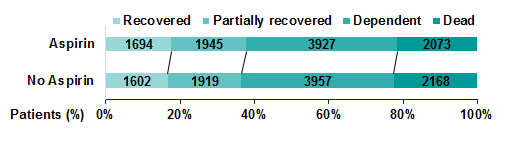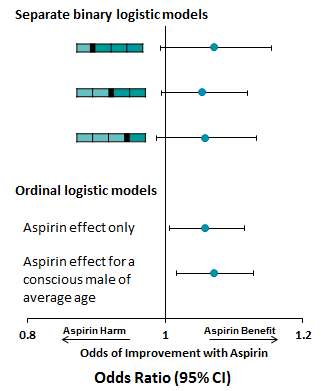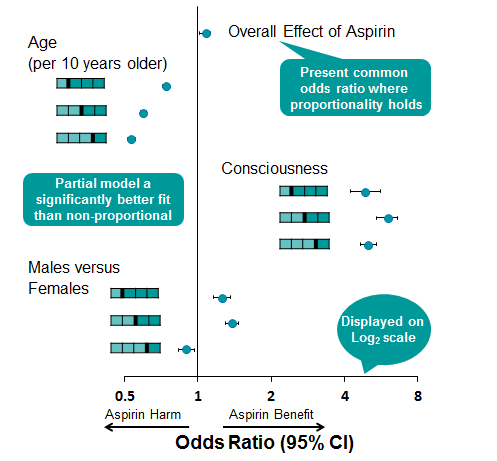<img alt="" src="https://secure.perk0mean.com/171547.png" style="display:none;">

# Understanding the Proportional Odds Assumption in Clinical TrialsOrdinal scales are commonly used to assess clinical outcomes; however, the choice of analysis is often sub-optimal.  In 2007, the Optimising Analysis of Stroke Trials (OAST) collaboration showed that ordinal-appropriate analyses of ordinal stroke outcome scales were preferable over binary analysis of a chosen ‘favourable’ outcome but uptake of ordinal methods between 2007 and 2014 has been low .

One barrier to uptake of ordinal methods might be the understanding and validation of the assumption of proportional odds.  We have presented an ordinal analysis of the effect of aspirin from the International Stroke Trial (IST), a large randomised study of 19,285 individuals, using SAS 9.3 to highlight the advantages and pitfalls of ordinal logistic regression where there may be doubt in the strength of the proportional odds assumption.

Ordinal Scale
Physical ability and dependency on care is assessed at six months following a stroke event, typically using an ordinal scale of ordered categories ranging from complete or partial recovery to dependency and death.  From Figure 1, we can see that a slight shift towards the lower scores and away from higher scores in individuals treated with aspirin in the IST.

Figure 1:Using a binary logistic model, we can see from Figure 2 that a small effect of aspirin is observed, however, the effect is not significant no matter the chosen partition of the outcome scale.

Performing ordinal logistic regression, we can produce a common odds ratio, which has a narrower confidence interval, suggesting this method has greater power to detect a significant effect, although this method is performed under the assumption of proportional odds.

The proportional odds assumption means that for each term included in the model, the 'slope' estimate between each pair of outcomes across two response levels are assumed to be the same regardless of which partition we consider.  A test of the proportional odds assumption for the aspirin term indicates that this assumption is upheld (p=0.898).

A potential pitfall is that the proportional odds assumption continues to apply when additional parameters are included in the model.  Guidelines from the Committee for Medicinal Products for Human Use (CHMP) published in 2013  recommend using adjusted analyses which include baseline covariates significantly related to the outcome.  Related covariates typically improve the fit of the model, however, in this case adding age, sex and consciousness on admission to hospital to the model causes the proportional odds assumption to be rejected (p<0.001). This means the assumption of proportional odds is not upheld for all covariates now included in the model.

Figure 2Benefits of Ordinal Logistic Regression - Exploring Proportionality of Data
In SAS version 9.3 or higher, options now exist to better explore the proportionality of your data using PROC logistic.  Specifying ‘unequalslopes’ removes the assumption that coefficients are equal between categories and instead produces an estimate for each model term at each partition of the scale.  The results can be viewed in Table 1.

PROC logistic data = asp_data order=internal outest=varlabels;
class asp conscious sex / param = ref;

/* Specify unequal slopes to obtain estimates for each model term at each partition of the outcome scale */
model score = asp age conscious sex / unequalslopes;
RUN;

Table 1:

 Analysis of Maximum Likelihood Estimates Parameter Estimate 95% Wald CL Pr > ChiSq Aspirin 1 1.075 0.996, 1.161 0.0650 2 1.068 1.003, 1.137 0.0399 3 1.073 0.996, 1.155 0.0619 Age (per 10 yrs older) 1 0.737 0.714, 0.762 <.0001 2 0.597 0.580, 0.615 <.0001 3 0.533 0.513, 0.555 <.0001 Conscious 1 4.878 4.225, 5.632 <.0001 2 5.982 5.411, 6.613 <.0001 3 5.010 4.637, 5.412 <.0001 Males versus Females 1 1.260 1.164, 1.365 <.0001 2 1.378 1.292, 1.470 <.0001 3 0.899 0.833, 0.970 0.0063

These test statements can be included under the model statement to test the proportional odds assumption for each covariate of the model.  The results of these tests can be seen in Table 2

/* Test partition estimates */

Aspirin:   test asp1_1 = asp1_2 = asp1_3;
Age:       test age_1 = age_2 = age_3;
Conscious: test conscious1_1 = conscious1_2 =conscious1_3;
Sex:       test sex1_1 = sex1_2 = sex1_3;
RUN;

Table 2

 Linear Hypotheses Testing Results Label WaldChi-Square DF Pr > ChiSq Aspirin 0.0484 2 0.9761 Age (per 10 years older) 237.8197 2 <.0001 Conscious 21.5721 2 <.0001 Males vs. Females 101.7181 2 <.0001

Table 1 shows us that the effect of aspirin is roughly constant over the scale and the hypothesis test in Table 2 indicates that the assumption of proportional odds holds for this parameter.  We can see that you are less likely to improve with each 10 years of age and that improvement becomes even less likely with each increase in score on the outcome scale and thus the proportional odds assumption does not hold for this parameter.  Similarly, the effect of consciousness is not constant across the scale, shown by rejection of the hypothesis test, however, being conscious upon admission to hospital confers significant benefit to your recovery after six months. Males were observed to have lower scores than females in the lower score categories but being male was observed to confer greater risk of death overall and consequently does not uphold the assumption of proportional odds.

Presenting a Partially Proportional Model
The proportionality restriction can be relaxed within the PROC logistic procedure for only those covariates not meeting the assumption.  In this case, the model statement can be modified to specify unequal slopes for age, consciousness and sex using the following syntax.  Figure 3 shows graphically the model estimates obtained from a partially proportional model, while a likelihood ratio test revealed that this model fitted significantly better than a fully non-proportional model.  The advantage of the partial proportional model is that a common estimate for aspirin can be obtained, while non-proportional parameters are not constrained.

model score = asp age conscious sex
/ unequalslopes=(age conscious sex);

Figure 3:Conclusion
By using PROC logistic to perform an ordinal logistic regression model, we have produced a more efficient estimate of the effect of aspirin and have several tools to explore the proportionality of data and adjust the proportionality restriction for only those covariates where the assumption is not upheld.  The pitfalls in using this type of model are that potential treatment harm can be masked by a single common odds estimate where the data have not been fully explored.This article was originally presented as a Quanticate poster titled 'Advantages and Pitfalls of Ordinal Logistic Regression' by our statistical consultancy group at the annual PSI ‘Promoting Statistical Insight and Collaboration in Drug Development’ conference in Berlin, Germany in May 2016. Learn more about how our team could support your clinical trial by scheduling a call with one of our sales representatives.

References

1. Optimising Analysis of Stroke Trials (OAST) Collaboration (2007) Can we improve the statistical analysis of stroke trials? Statistical reanalysis of functional outcomes in stroke trials. Stroke. 38(6): 1911-5.
2. Nunn et al. (2016) Analysis of the Modified Rankin Scale in Randomised Controlled Trials of Acute Ischaemic Stroke: A Systematic Review. Stroke Research and Treatment. Vol 2016: Article ID 9482876.
3. International Stroke Trial Collaborative Group (1997) The International Stroke Trial (IST): a randomised trial of aspirin, subcutaneous heparin, both, or neither among 19 435 patients with acute ischaemic stroke. Lancet, Volume 349, No. 9065, p1569–1581.
4. Committee for Medicinal Products for Human Use (CHMP) (2013) Guideline on adjustment for baseline covariates in clinical trials. EMA/CHMP/295050/2013.

## Related Articles#### Re-Randomization Tests in Equivalence Trials: Can We Still Use Them?

by Statistical Consultancy Team#### Estimands – What you need to know

by Statistical Consultancy Team